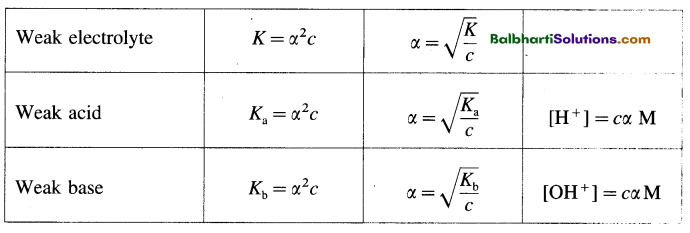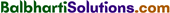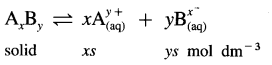By going through these Maharashtra State Board 12th Science Chemistry Notes Chapter 3 Ionic Equilibria students can recall all the concepts quickly.

## Maharashtra State Board 12th Chemistry Notes Chapter 3 Ionic Equilibria

→ Formulae on Ostwald’s dilution law:→ Kw = [H3O+] × [OH], at 25°C Kw = 1 × 10-14

→ pH = -log10[H+]; pOH = -log10[OH]→ pH + pOH = 14

→ Acidic buffer solution: pH = PKa + log10 $$\frac { [salt] }{ [acid] }$$

→ Basic buffer solution: pOH = pKb + log10 $$\frac { [salt] }{ [acid] }$$

→ PKa = – log10 Ka; pKb = – log10 Kb

→ Solubility product:ksp = (xs)x (ys)y = xxyy x (S)x+y